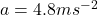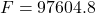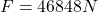## How much force is needed to accelerate a 9,760 kg airplane at a rate of 4.8 m/s2?

Question

How much force is needed to accelerate a 9,760 kg airplane at a rate of 4.8 m/s2?

in progress 0
6 months 2021-08-07T15:36:03+00:00 1 Answers 7 views 0

46848N

Step – by – step explanation:

We can calculate the force needed to accelerate the airplane using the relation, F =ma, where

F = Force

m= mass

a= acceleration

Given that,

m=9,760 kg, andBy substitution,This implies that,Hence the force needed to accelerate the airplane is 46848N